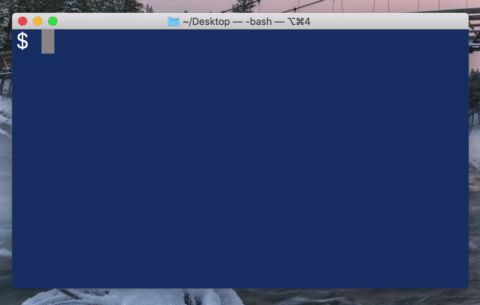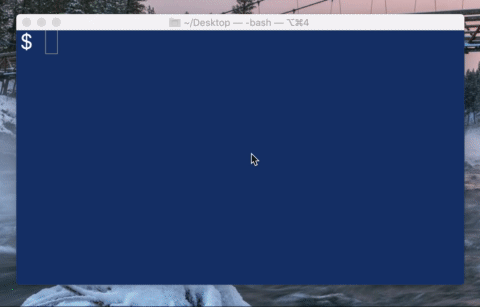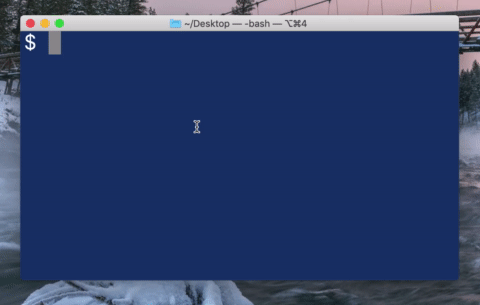A couple months ago I wrote a script that needed to take a CSV file with ~500k lines and process records from each of those lines. I wanted some way to keep track of the progress, and logging out each line number on a separate line was filling my console really fast. Even just outputting a single period without a newline was overwhelming. This led to me researching how to overwrite a console line. I decided to make a quick video about how to do it and try to share my knowledge.

## The IO Module

If you’ve been working with Elixir for very long, you’ve probably seen `IO.inspect/2`. You can add it to your pipelines to be able to easily debug output at various stages of processing. `IO.inspect/2` is built on top of `IO.puts/1` which you may also be familiar with. Basically `IO.puts/1` prints whatever you tell it to in the console on a new line. This can be pretty useful for debugging output as well.

What I’m excited to show you today is `IO.write/2`. I’ve been working primarily in Elixir for two years now, but somehow I had still never used `IO.write/2` until pretty recently. `IO.write/2` gives you the ability to print onto the same line as your last output, rather than putting it on a new line. This allows for some pretty cool tricks.

## Tracking TimeTo start with, let’s create a simple clock that will run in your terminal. You can see the full code below, but we’ll create a `Clock` module and create a function inside of it simply called `start`. This function will call `Time.utc_now()` to get the current UTC time. Then we’ll tell it that we only care about seconds, so we’ll use `Time.truncate/2` to discard any millisecond and microsecond data. Finally we’ll convert the time struct to a string.

``````defmodule Clock do
def start do
Time.utc_now()
|> Time.truncate(:second)
|> Time.to_string()
end
end
``````

Now here’s where `IO.write/2` comes in. We’ll write the string out to the terminal. Then we’ll use `Process.sleep/1` to tell the process to sleep for 1 second until it’s ready to display the next time. Then we’ll recursively call this same function so that it will create the loop that checks the clock every second. Finally we’ll run our `Clock.start/0` function outside of the module. And now let’s run this in our terminal.

``````defmodule Clock do
def start do
...
IO.write(time_string)
Process.sleep(1_000)
start()
end
end

Clock.start()
``````

You’ll notice that now it’s printing the current time on the same line as the previous one, but we really want it to replace the current time instead. This is where special characters come in. You’ve likely seen the character for a newline (`/n`). Maybe you’ve seen the tab character as well (`/t`). I’m guessing it’s likely though that you haven’t seen the character for a carriage return. `/r` is a carriage return, and it returns the cursor to the beginning of the current line. When you use it you can replace text on the current line. So we’ll add that and then our final code looks like:

``````defmodule Clock do
def start do
time_string =
Time.utc_now()
|> Time.truncate(:second)
|> Time.to_string()

IO.write("\r#{time_string}")

Process.sleep(1_000)
start()
end
end

Clock.start()
``````

## Counting Down to the EndSo now let’s try a different application. We’ll make a countdown: it will start at 10 and work it’s way down to 0. When it reaches 0, we’ll print out that it’s done. So for this, let’s make a module called `Count` with a function called `count` that takes in the current number. Let’s make that function write the current number to the terminal line, using our carriage return character to overwrite what was already there. Then we’ll recursively call it again with the decremented count. To slow things down and make it more obvious what’s going on, I’m going to use the `Process.sleep/1` function again. Now we need to handle the base case as well and tell the program to stop when it hits 0, so let’s use pattern matching to stop when the count is at 0. And finally let’s run that function when this file gets run. Your code will look something like this:

``````defmodule Count do
def count(0) do
IO.puts("\nDone!")
end

def count(current) do
IO.write("\r#{current}")
Process.sleep(250)
count(current - 1)
end
end

Count.count(10)
``````

Now if you try running that, you’ll notice something strange. The 0 from the 10 never goes away. That’s because when we use the carriage return, we’re telling it to overwrite what’s already there, but only as far as we actually write over it. If we don’t supply a character to replace the one that’s already there, it will leave it. So we’re going to need to add some blank spaces to overwrite the end characters. Since this is simply a countdown from 10 we can just add a single space to the end, but if it were to allow users to input whatever value they wanted, we would need to programmatically infer how many spaces to add. So if we run that again with the code below, it does what we expect.

``````defmodule Count do
def count(0) do
IO.puts("\nDone!")
end

def count(current) do
IO.write("\r#{current} ")
Process.sleep(250)
count(current - 1)
end
end

Count.count(10)
``````

## Great ProgressThat’s most of what I wanted to show you, but I want to end by showing you one of the coolest applications for this. This example is a little bit more difficult to follow, but it will allow you to add a simple progress bar to a CLI tool. My friend Trevor Fenn implemented it in Ruby for some stuff we’re doing at Podium, so I’ve ported it over to Elixir for this example.

To start off we’re going to make a module called `Progress` containing a function called `bar`. Let’s have `bar` receive two parameters: `count` and `total`. I’m going to add a private `percent_complete` function that takes in the same `count` and `total` and we’ll calculate the actual percentage here. So let’s start by dividing the count by the total which gives us the decimal percentage, and then we’ll multiply that by 100 to give us a more human readable percentage. Then finally let’s round it to the nearest hundredth so that it doesn’t get too big. I’m going to use a module attribute constant for the rounding precision though so that it’s easily changeable if we want to do that later.

``````defmodule Progress do
@rounding_precision 2

def bar(count, total) do
end

defp percent_complete(count, total) do
Float.round(100.0 * count / total, @rounding_precision)
end
end
``````

OK now that we have the percentage complete, we need to actually create the progress bar from that. So how this progress bar will work is we’re going to have two different unicode characters that we’re using. The first is a halftone box (░) and the other is a filled box (█). We’ll want to show the filled box the number of times that it takes to show the current progress and then the halftone box for the rest of it. So I’m going to create another module attribute constant called `@progress_bar_size` to say how many characters long the progress bar should be. I think I’m going to say 50 for now to simplify the math.

``````@progress_bar_size 50
``````

So to figure out how many percentage point each character is worth I will divide 100 by the progress bar size, so in this case, each character is worth 2% of the total. Then we’ll calculate how many completed characters we should write to the line and then subtract that from the total progress bar size to know how many incomplete characters to write.

``````def bar(count, total) do
percent = percent_complete(count, total)
divisor = 100 / @progress_bar_size

complete_count = round(percent / divisor)
incomplete_count = @progress_bar_size - complete_count
end
``````

In the video, I created a new function called `repeat` that returns a string with the specified number of that character. When I showed my code to Trevor though, he showed me that there is a `String.duplicate/2` that will do exactly what I was going for, so we’ll use that to generate the full progress bar. We’ll start by writing the repeated completed characters and then we’ll append the incomplete characters onto that. And finally let’s write the percent complete at the end to make it a little more usable.

``````defmodule Progress do
...
@complete_character "█"
@incomplete_character "░"
def bar(count, total) do
...
complete = String.duplicate(@complete_character, complete_count)
incomplete = String.duplicate(@incomplete_character, incomplete_count)

"#{complete}#{incomplete}  (#{percent}%)"
end
``````

So now let’s actually run it. Outside of the module let’s say we have a total of 50 tasks to complete. So we’ll call `Enum.each` over a range from 1 to 50 and with each number we can generate a progress bar with the current task number and the total. Let’s go ahead and write this to the console with a carriage return at the front to tell it to overwrite the previous progress bar. Then to make it a little more visible, let’s add a `Process.sleep/1` to each iteration. And then at the end let’s write a newline so that when the execution finishes it moves our cursor down to the next line.

``````defmodule Progress do
...
end

total = 50

Process.sleep(50)
end)

IO.write("\n")
``````

So overall, you’re looking at something like this.

``````defmodule Progress do
@rounding_precision 2
@progress_bar_size 50
@complete_character "█"
@incomplete_character "░"

def bar(count, total) do
percent = percent_complete(count, total)
divisor = 100 / @progress_bar_size

complete_count = round(percent / divisor)
incomplete_count = @progress_bar_size - complete_count

complete = String.duplicate(@complete_character, complete_count)
incomplete = String.duplicate(@incomplete_character, incomplete_count)

"#{complete}#{incomplete}  (#{percent}%)"
end

defp percent_complete(count, total) do
Float.round(100.0 * count / total, @rounding_precision)
end
end

total = 50